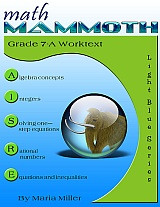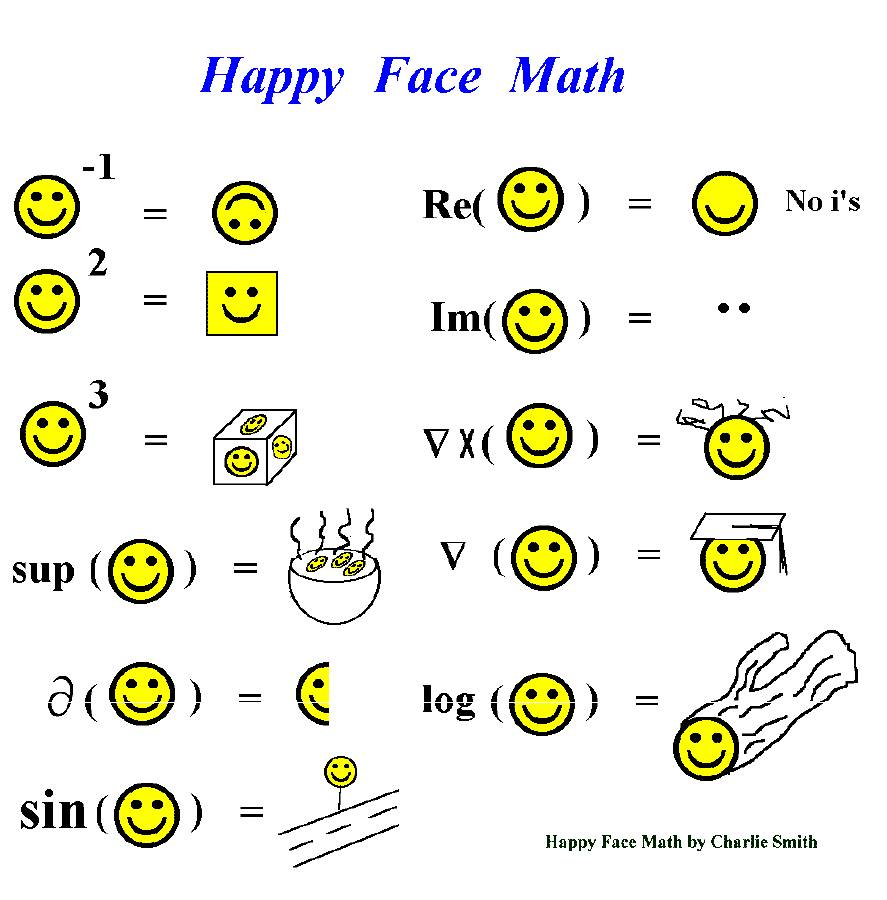Maria's Math News, February 2015
 Hello! This time we explore how students should show their work, laugh at "Happy Face Math", get tips for writing in math class, and celebrate: ALL worksheets at HomeschoolMath.net can now be made both in PDF and html formats!~Maria Math Mammoth news How should students show their work for word problems? (grades 3-8) Math worksheets in PDF form (grades 1-9) Writing in math class (grades 4-12) Happy Face Math! (grades 5-12) Trouble viewing this email?   See it online →

## 1. Math Mammoth news

1. I've just updated the page where I collect comparisons between Math Mammoth and other math curricula. I added to it a section on CLE math and a user story someone just sent me on Math Mammoth vs. Horizons.

Feel free to use the form on that page to send me YOUR comparison of MM vs. some other math curriculum.
2.Are you one of those who would like to start Math Mammoth Grade 7-B now? Like I have mentioned in the past, since 7-B is not available yet, we are including the lessons from the proportions chapter of 7-B in the corresponding Blue series book. You can get that book to "keep you going" for now -- it should be ready in just a day or two.

If you've purchased it (either separately or as part of a Blue series bundle) in the past, you can get the updated version for free. If you need to purchase it for this purpose, I will reimburse you for that amount from the price of 7-B when it comes available.

## 2. How should students show their work for math word problems?

Personally, in the lower grades, I'd ask the child to EXPLAIN their thought processes orally, and then gradually teach them to write something on paper. The main thing students in grades 1-3 need to write is the actual calculations they did, not only the final answer.

For example, if they added 23 and 87 to get the answer, they should write 23 + 87 = 110 and include the units of whatever it was, such as \$23 + \$87 = \$110 or 23 cm + 87 cm = 110 cm.

In the upper elementary grades (4-6) I'd like to see students write sentences and/or words in addition to the calculations so that another person can follow their solution.

I'll give some examples.

Mr. Jefferson travels from Paducah to Lexington and back, three times a month. What is his total mileage? (A map shows that the distance in question is 255 miles.)

An example solution showing the work:

One round trip is 255 + 255 = 510 miles.
Three round trips are 510 + 510 + 510 = 1,530 miles.

Mick earned \$345 from strawberry picking, and Jeanine earned three times as much. How much did they earn in total?

A concise solution showing the work:

Jeanine: 3 × \$345 = \$1,035
Total: \$345 + \$1,035 = \$1,380

A bit more wordy solution showing the work:

Jeanine earned 3 × \$345 = \$1,035.
In total, Mick and Jeanine earned \$345 + \$1,035 = \$1,380

Both should allow another person to follow the reasoning.

A T-shirt cost \$10.50, but now it is discounted by 2/5 of its price.
Annie buys ten shirts with the discounted price. What is her total bill?

An example concise solution showing the work:

Cost of one shirt:
\$10.50 ÷ 5 = \$2.10
3 × \$2.10 = \$6.30

Total bill:
10 × \$6.30 = \$63.00

A bit more wordy solution:

One shirt costs 3/5 of \$10.50, which is \$10.50 / 5 × 3 = \$6.30.
Then ten shirts cost 10 × \$6.30 = \$63.00.

David pays a 20% income tax on his \$2,100 salary.
a. How many dollars is the tax?
b. How much money does he have left after paying the tax?

These are very simple questions so this time writing the calculations is enough.

a. \$2,100 / 10 = \$210
2 × \$210 = \$420

or \$2,100 / 5 = \$420.

b. Again, all that's necessary to show the work is to write
a subtraction: \$2,100 − \$420 = \$1,680

14. A herd of 40 horses had some bay, some chestnut, and some white horses. Thirty percent of them are bay, and 45% are chestnut. How many horses are white?

An example solution showing the work:

100% − 30% − 45% = 25%.
So 25% of the horses are white.
25% is 1/4.
And 1/4 of 40 horses is 10 horses.

OR

Percentage of white horses:
100% − 30% − 45% = 25%
25% of 40 horses is 10 horses.
So 10 horses are white.

In a nutshell:

The purpose of writing down the work allows someone else to follow the person's thought processes. This is of course important for students to learn no matter what their future occupation: they need to be able to explain to others how they solve a problem, whether a math problem or a problem in some other field of life!

One more tip: You could ask a fellow student or sibling to read the student's work to check if it can be followed and understood!

## 3. Math worksheets in PDF form

Time to celebrate!

I've been working hard on the various worksheet generators at HomeschoolMath.net, and now all of them make worksheets both in PDF and html formats!

Below you can see examples of the variety of the worksheets:

The advantage of the PDF format is that it's portable and you'll instantly see how it prints.

The advantage of the html format is that you can edit it! Simply save the worksheet to your device and then open the file in a word processing software.

The worksheets cover topics from grade 1 through pre-algebra, such as: all the basic operations, clock, money, measuring, fractions, decimals, percents, proportions, ratios, factoring, equations, expressions, graphing, geometry, square roots, and more. And it's all free.

=> Free math worksheets

Enjoy!

## 4. Writing in math class

I really liked these little writing tasks meant for the beginning of lesson, but you could use them any time, and of course they're not limited to algebra! Including writing in math is something I'm trying to do from time to time with my girls by simply asking them to write out a neat solution for a word problem - these are even better.=> Incorporating writing in math class

## 5. Happy Face Math(image credit Charlie Smith www4.stat.ncsu.edu/~bmasmith/funstuff.html)

Some pointers in case you find these cryptic:

• A happy face to the power of -1 is the inverse of happy face.
• Happy face squared or cubed are pretty easy.
• "sup" in mathematics is the supremum of a set.
• Then we have a partial derivative of Happy Face.
• Next, sine of Happy Face = Happy Face sign by the road.

• In the second column, Re() is the real part of Happy
• Face, which is Happy Face without the eyes (without i's).
• Im of Happy Face is the imaginary part of Happy Face = the eyes (the i's).
• [Note, i is the imaginary unit used with imaginary numbers.]

• Triangle followed by X is the mathematical operation curl (of a vector).
• Next, the upside-down triangle is the gradient of Happy Face or "grad" of Happy Face.
• Lastly, Log or logarithm of Happy Face

•  That's it this time! I hope you found something helpful.Feel free to forward this issue to a friend/colleague! Subscribe here. Till next month, Maria Miller

--